#Function Repository Resource:

# ShortestTourArt3D

Display a 3D model using a continuous line

Contributed by: Enrique Zeleny
 ResourceFunction["ShortestTourArt3D"][model3D] takes the points from model3D, finds the shortest path between these points and plots a continuous line for this path. ResourceFunction["ShortestTourArt3D"][model3D,n] only takes n points from model3D to plot the line that connects the points.

## Details and Options

FindShortestTour is effectively used to find the shortest path.

## Examples

### Basic Examples

Show shortest path lines connecting vertices in 3D graphics:

 In:=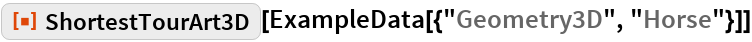Out=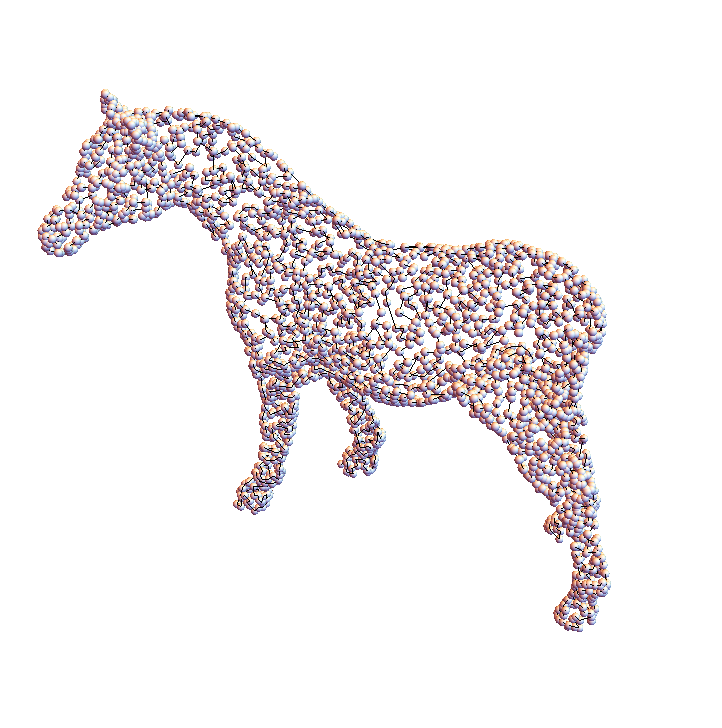Show only 3000 points with the original 3D model:

 In:=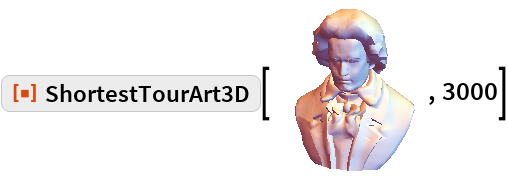Out=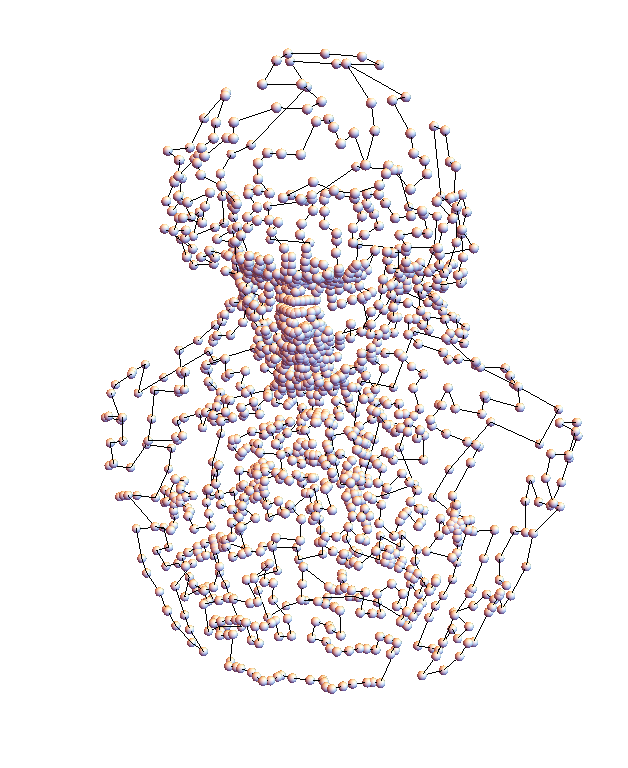In:=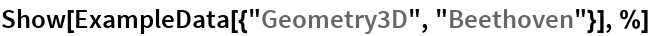Out=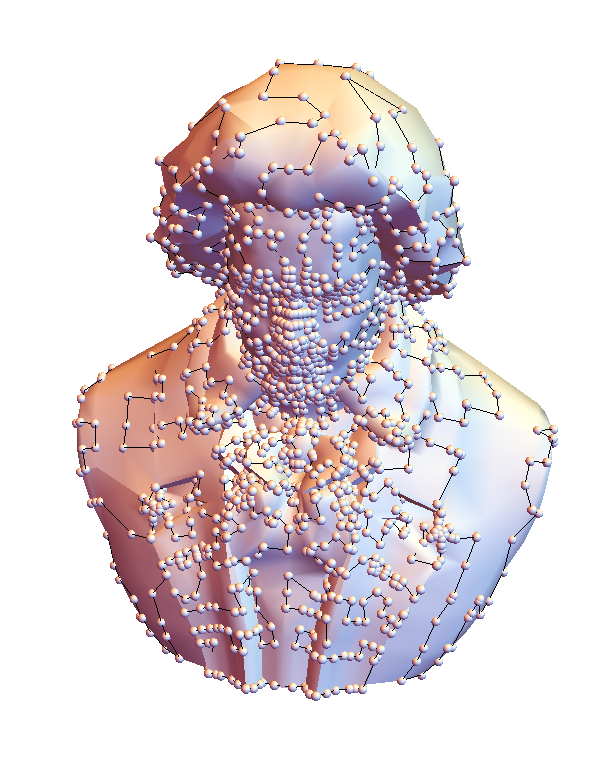Generate an animation:

 In:=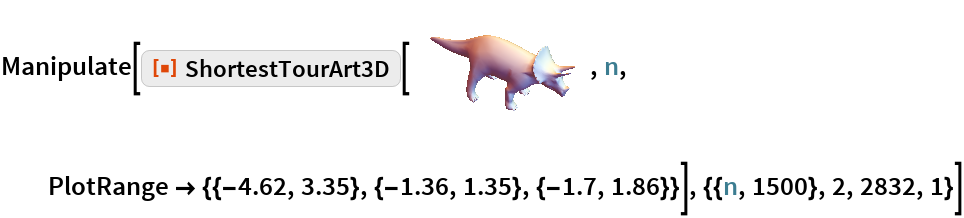Out=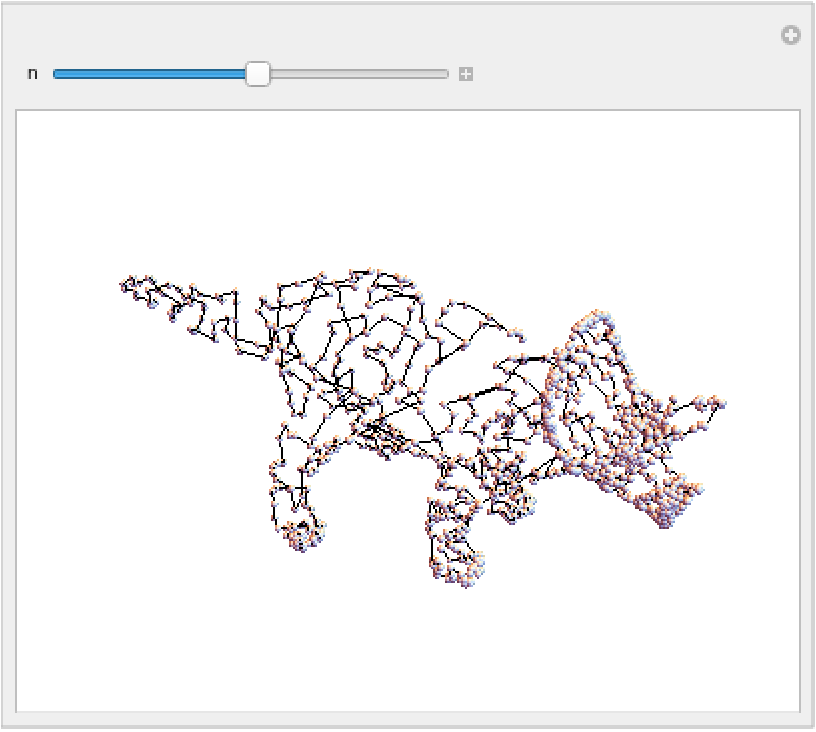### Scope

Works for other types of 3D graphics that contain a GraphicsComplex:

 In:=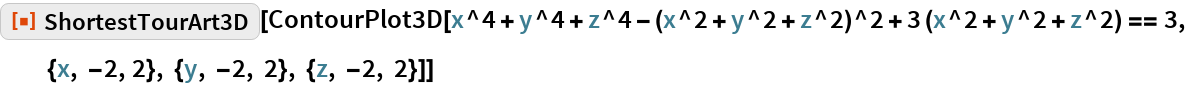Out=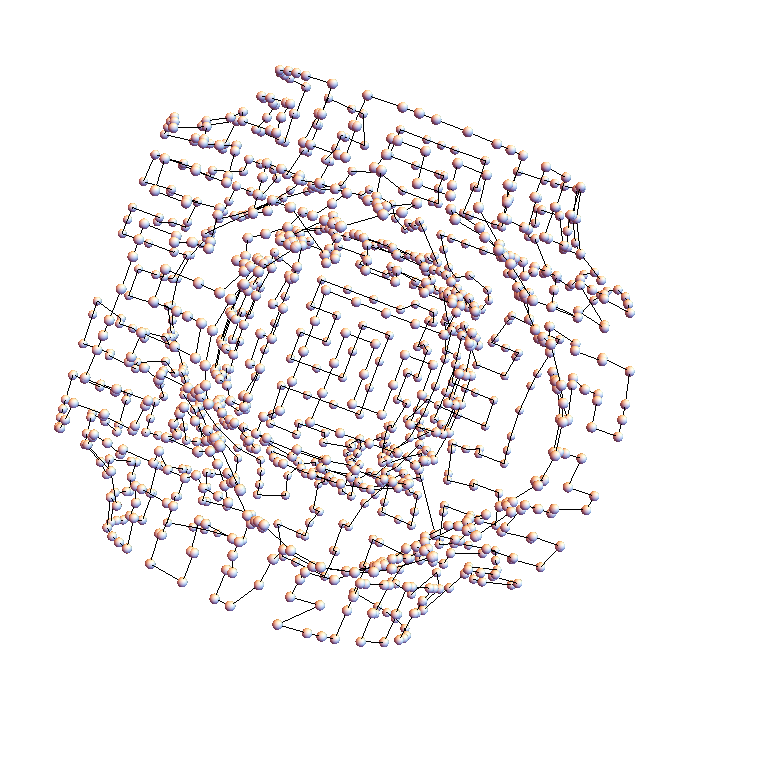## Requirements

Wolfram Language 11.3 (March 2018) or above